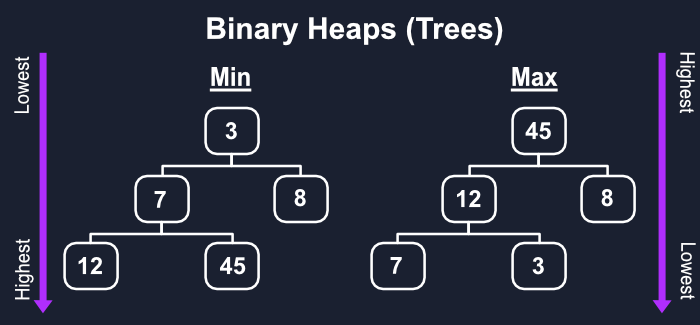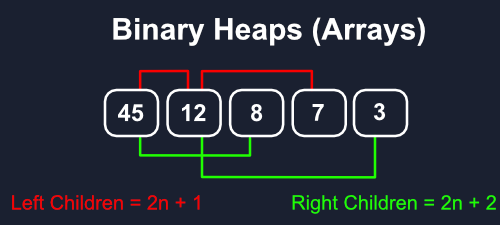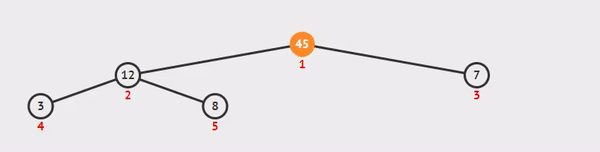# Binary Heaps and Priority Queues via JavaScript

Published on April 5, 2020By Joshua Hall
Developer and author at DigitalOcean.While we believe that this content benefits our community, we have not yet thoroughly reviewed it. If you have any suggestions for improvements, please let us know by clicking the “report an issue“ button at the bottom of the tutorial.

While I’m sure we can all agree that queues are the coolest things since sliced bread, we can actually do much better by mixing them with a variation of trees called heaps. With heaps we can structure our data into a more intelligent queue that’s sorted by importance rather than order.

## Concepts

Unlike with binary search trees, where we compared and organized our values across siblings, with heaps we only work between parents and their children. This gives us two possibilities for heaps, the `max heap` and the `min heap`, for whether you’re moving from the highest to the lowest values or vice versa. For simplicity’s sake, we’re only going to focus on the max heap, since it’s so easy to convert it to a min heap.

Like with binary search trees, binary heaps are only allowed to have two or fewer children to a parent. They are also special since they are always balanced because every new node will be added to a level from left to right until full.Sadly, linked-lists generally aren’t the best approach for binary heaps, despite being usually conceptualized as a tree with left and right children, although it’s still possible.

Most of the time it’s going to be better to handle it as an array, so that’s what we’re going to cover here. The order is as you’d expect with everything left to right on a level before moving to the next level.

This way, we create a very consistent pattern for finding a node’s children. All of a node’s left children will be exactly at a position `2n + 1` away from their parent, with `n` being their parent’s index, and all right children being `2n + 2`.It would seem like adding a new node would be as simple as pushing onto our array, but the tricky part is that we need to compare it with the parents in between itself and the max, then re-order them accordingly.Graphic/Animation thanks to VisuAlgo.net

After we push our new item onto the end of the array we’ll need to “bubble up” our larger values. First, we need to grab the new item at the end of the array, which we’ll break into the index and the new item at that index.

Every time we add an item we’re going to use the reverse of our equation for finding children, `(n-1)/2`, to find its parent. If its parent is less than the current node, swap them then save its index which will be the next `current`. This will continue until there are no parents left.

Since it will gradually be moving our `index` up from the end, as long as it’s greater than zero, keep swapping.

``````class BH {
constructor() {
this.values = [];
}
this.values.push(element);
let index = this.values.length - 1;
const current = this.values[index];

while (index > 0) {
let parentIndex = Math.floor((index - 1) / 2);
let parent = this.values[parentIndex];

if (parent <= current) {
this.values[parentIndex] = current;
this.values[index] = parent;
index = parentIndex;
} else break;
}
}
}

const tree = new BH();
console.log(tree); // [31, 6, 4, 3]
``````

## Remove Max

Removing the topmost node is a bit more complicated than you would think. We’re going to return the first node, our max, then take the last node, the end of our array, and set that as the new max.

We do that so we can use our lowest value as an easy baseline to compare with our other values as we “sink down” back to the bottom of the tree while making our comparisons and swaps along the way.Graphic/Animation thanks to VisuAlgo.net

The simple part is grabbing our current max value and popping it off before replacing it with the last item, then we can return our original max value after everything else is done.

Once we have a starting index, we want to grab both its right and left children. If the left child is a valid item and is larger, then we can save it as `swap` to run the swap when all the comparisons are done.

The right child is a bit more complicated, we only want one, and the largest, child to be swapped with the parent. We’ll add a separate requirement that `rightChild` can only be set as `swap` if it hasn’t been defined yet or it’s larger than `leftChild`.

``````class BH {
extractMax() {
const max = this.values;
const end = this.values.pop();
this.values = end;

let index = 0;
const length = this.values.length;
const current = this.values;
while (true) {
let leftChildIndex = 2 * index + 1;
let rightChildIndex = 2 * index + 2;
let leftChild, rightChild;
let swap = null;

if (leftChildIndex < length) {
leftChild = this.values[leftChildIndex];
if (leftChild > current) swap = leftChildIndex;
}
if (rightChildIndex < length) {
rightChild = this.values[rightChildIndex];
if (
(swap === null && rightChild > current) ||
(swap !== null && rightChild > leftChild)
)
swap = rightChildIndex;
}

if (swap === null) break;
this.values[index] = this.values[swap];
this.values[swap] = current;
index = swap;
}

return max;
}
}

const tree = new BH();
console.log(tree.extractMax()); // 31
``````

## Priority Queues

With a few minor tweaks we can mix binary heaps with queues and create a type of queue that organizes our data by importance rather than when it was added.

We can achieve this simply enough by storing nodes instead of single numbers. Each node will have a priority level (let’s say from 1-5), which it will use to determine the order. When the priorities on two nodes are the same, the left child, since it will have been added first, will go first.

All we have to do is use the node’s `priority` every time we make a comparison in an `if` statement.

``````class Node {
constructor(val, priority) {
this.val = val;
this.priority = priority;
}
}

class PQ {
constructor() {
this.values = [];
}
enqueue(val, priority) {
let newNode = new Node(val, priority);
this.values.push(newNode);
let index = this.values.length - 1;
const current = this.values[index];

while (index > 0) {
let parentIndex = Math.floor((index - 1) / 2);
let parent = this.values[parentIndex];

if (parent.priority <= current.priority) {
this.values[parentIndex] = current;
this.values[index] = parent;
index = parentIndex;
} else break;
}
}
dequeue() {
const max = this.values;
const end = this.values.pop();
this.values = end;

let index = 0;
const length = this.values.length;
const current = this.values;
while (true) {
let leftChildIndex = 2 * index + 1;
let rightChildIndex = 2 * index + 2;
let leftChild, rightChild;
let swap = null;

if (leftChildIndex < length) {
leftChild = this.values[leftChildIndex];
if (leftChild.priority > current.priority) swap = leftChildIndex;
}
if (rightChildIndex < length) {
rightChild = this.values[rightChildIndex];
if (
(swap === null && rightChild.priority > current.priority) ||
(swap !== null && rightChild.priority > leftChild.priority)
)
swap = rightChildIndex;
}

if (swap === null) break;
this.values[index] = this.values[swap];
this.values[swap] = current;
index = swap;
}

return max;
}
}

const tree = new BH();
tree.enqueue(3, 2);
tree.enqueue(4, 5);
tree.enqueue(31, 1);
tree.enqueue(6, 3);
console.log(tree.dequeue()); // 4
console.log(tree.dequeue()); // 6
console.log(tree.dequeue()); // 3
console.log(tree.dequeue()); // 31
``````

## Closing Thoughts

Just like how we used standard queues for tree traversal priority queues will be essential for intelligently traversing graphs and more complicated structures.

Converting a max heap to a min heap is as simple as changing our greater than to less than sign in all of our comparisons.Developer and author at DigitalOcean.

#### Still looking for an answer?

You can type !ref in this text area to quickly search our full set of tutorials, documentation & marketplace offerings and insert the link!

Can you please share some background info on this?

• Is this about data-structure & algorithm? • How do the following differ? : binary, binary tree, binary search tree, heap, tree. • Can you please share a practical application of the concepts discussed here? • I guess I can imagine it’s application in Backend, however, I’m unable to figure it’s relevance in Front-end web development. Can you please help?

I think I need to learn some background of this article, the concepts discussed.